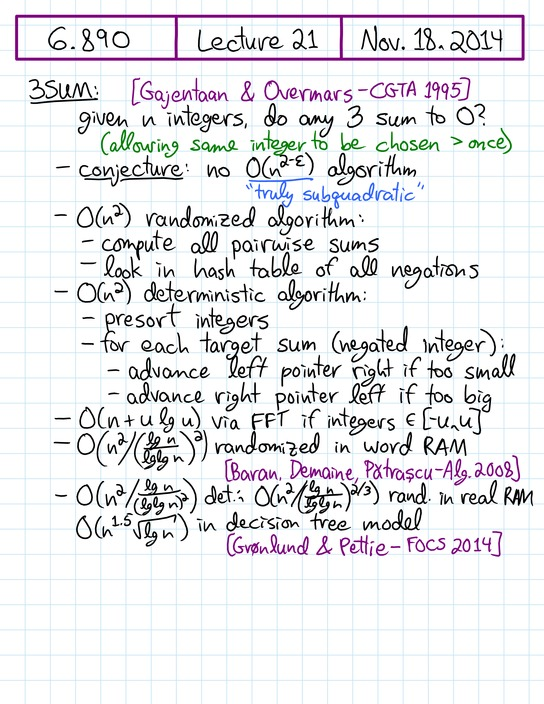6.890 Algorithmic Lower Bounds: Fun with Hardness Proofs (Fall '14)

Prof. Erik Demaine     TAs: Sarah Eisenstat, Jayson Lynch

[Home] [Lectures] [Problem Sets] [Project] [Open Problems] [Piazza]

Lecture 21 Video     [previous] [next]

 [+] 3SUM & APSP. k-SUM upper and lower bounds, reductions. Distinct 3SUM, 3SUM′, GeomBase, 3 points on a line, point on 3 lines, Separator, Strips cover box, Triangles cover triangle, Hole in union, Triangle measure, Point covering, Visibility between segments, Visible triangle, Planar motion planning, 3D motion planning, fixed-angle chains. Nonquadratic lower bounds for triangle finding. Conjectured cubic graph problems: diameter, all-pairs shortest paths, negative triangle, radius, median. [Scribe Notes] [src] This lecture is about hardness of problems that can be solved in polynomial time but where that polynomial seems to be substantially superlinear. Our focus will be on 3SUM (given n integers, do any 3 sum to zero?) which is conjectured to require roughly Θ(n2) time to solve. It has been the basis for many “3SUM-hardness” reductions, so if any of the problems can be solved in O(n2 − ε) time, then so can 3SUM. In class, we'll prove many problems 3SUM-hard, including a lot of computational geometry (where 3SUM originally comes from). We'll also talk about the generalization k-SUM, which has fairly strong lower bounds assuming ETH, and the conjectured-cubic graph problems all-pairs shortest paths and diameter.
 No support for video detected. Install Flash or use an HTML5 browser. Download Video: 360p, 720p Handwritten notes, page 1/8 • [previous page] • [next page] • [PDF]Handwritten notes, page 1/8 • [previous page] • [next page] • [PDF] Slides, page 1/13 • [previous page] • [next page] • [PDF]Figure drawn by Erik Demaine based on Figure 11 of http://​dx.doi.org/​10.1016/​0925-7721(95)00022-2 Slides, page 1/13 • [previous page] • [next page] • [PDF]

The video above should play if your web browser supports either modern Flash or HTML5 video with H.264 or WebM codec. The handwritten notes and slides should advance automatically. If you have any trouble with playback, email Erik.